# 7.7: Acyl Groups, RCO-

$$\newcommand{\vecs}{\overset { \rightharpoonup} {\mathbf{#1}} }$$ $$\newcommand{\vecd}{\overset{-\!-\!\rightharpoonup}{\vphantom{a}\smash {#1}}}$$$$\newcommand{\id}{\mathrm{id}}$$ $$\newcommand{\Span}{\mathrm{span}}$$ $$\newcommand{\kernel}{\mathrm{null}\,}$$ $$\newcommand{\range}{\mathrm{range}\,}$$ $$\newcommand{\RealPart}{\mathrm{Re}}$$ $$\newcommand{\ImaginaryPart}{\mathrm{Im}}$$ $$\newcommand{\Argument}{\mathrm{Arg}}$$ $$\newcommand{\norm}{\| #1 \|}$$ $$\newcommand{\inner}{\langle #1, #2 \rangle}$$ $$\newcommand{\Span}{\mathrm{span}}$$ $$\newcommand{\id}{\mathrm{id}}$$ $$\newcommand{\Span}{\mathrm{span}}$$ $$\newcommand{\kernel}{\mathrm{null}\,}$$ $$\newcommand{\range}{\mathrm{range}\,}$$ $$\newcommand{\RealPart}{\mathrm{Re}}$$ $$\newcommand{\ImaginaryPart}{\mathrm{Im}}$$ $$\newcommand{\Argument}{\mathrm{Arg}}$$ $$\newcommand{\norm}{\| #1 \|}$$ $$\newcommand{\inner}{\langle #1, #2 \rangle}$$ $$\newcommand{\Span}{\mathrm{span}}$$$$\newcommand{\AA}{\unicode[.8,0]{x212B}}$$

1. The functionis called an acyl group and in specific cases in named by adding the suffix -oyl to the appropriate hydrocarbon prefix. That is, alkane- $$+$$ -oyl becomes alkanoyl: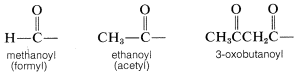Acyl groups also may be called alkanecarbonyl or cycloalkanecarbonyl groups:2. When an acyl group replaces the hydrogen of alcohols, carboxylic acids, hydrogen halides, ammonia or amines, we have the acyl compounds known as esters, anhydrides, halides, and amides, respectively.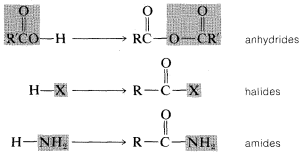Each of these types of compounds are named as follows.Image

## Carboxylic Esters, $$RCO_2R'$$

1. The name of the parent carboxylic acid (alkanoic) is changed to alkanoate and is preceded, as in a separate word, by the name of the ester alkyl group $$R'$$:2. When appropriate, esters also are named as carboxylates:3. When it is necessary to name the $$-CO_2R'$$ function as a substituent, it becomes alkoxycarbonyl,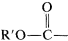. (Notice that this is structurally different from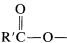.)Notice the use of parentheses to separate the numbering of $$C3$$ of the cyclohexane ring from the numbering of the chain.

4. It also may be necessary at times to name the $$R'CO_2-$$ group as a substituent, in which case it becomes acyloxy- or $$R'$$-carbonyloxy-. For example,## Carboxylic Anhydrides, $$RCOOCOR'$$

Symmetrical anhydrides ($$R=R'$$) are named after the parent acid; unsymmetrical or "mixed" anhydrides ($$R \neq R'$$) cite each of the parent acids: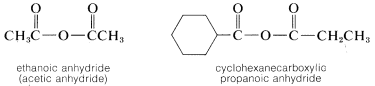## Acyl Halides, $$RCOX$$

The acyl group, $$RCO-$$, and the halogen (as halide) are cited separately:## Amides, $$RCONH_2$$

1. The suffix amide is appended to the name of the hydrocarbon corresponding to the carbon chain that includes the carbonyl group. That is, alkan(e) $$+$$ amide $$=$$ alkanamide. A one-carbon chain is a carboxamide:2. When the amide nitrogen is substituted with lower-ranking groups than the acyl group, the substituents are designated as prefixes. The letter N is used to show that the substitution is on nitrogen: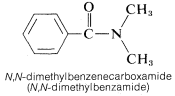3. Names for amides as substituents include the following: